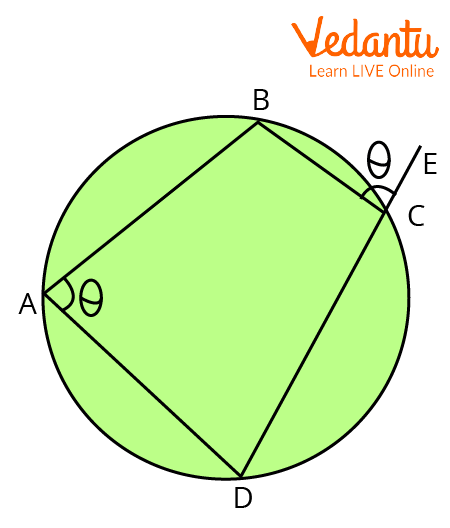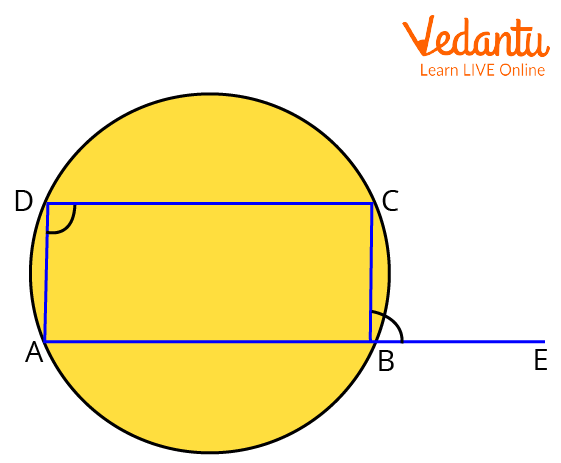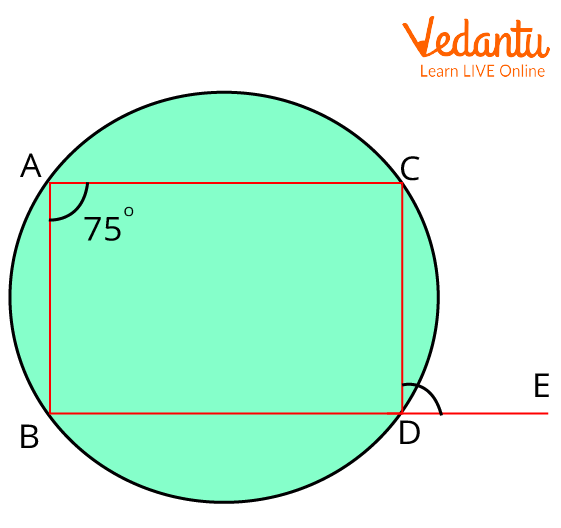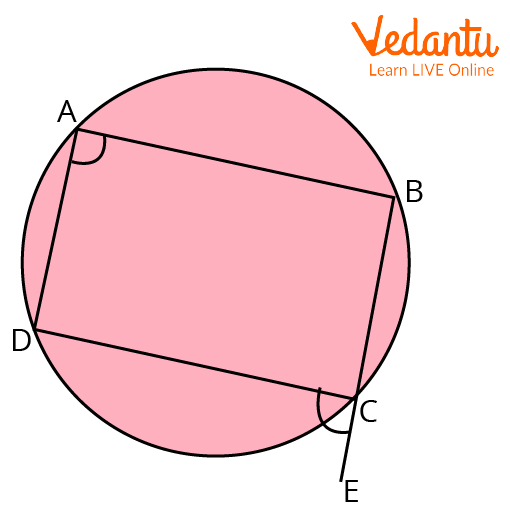Courses
Courses for Kids
Free study material
Free LIVE classes
More

# Theorem on the Exterior Angle of a Cyclic QuadrilateralLIVE
Join Vedantu’s FREE Mastercalss

## An Overview

The theorem on the exterior angle of a cyclic quadrilateral is used to state the property of cyclic quadrilaterals and helps us in solving complex problems related to exterior angles in an easy and efficient manner. Cyclic quadrilaterals are the quadrilaterals that are formed when all the vertices of the quadrilateral lie on the circumference of the circle. In this article, we will discuss the detailed proof of the theorem related to exterior angles of the cyclic quadrilateral along with the application of the cyclic quadrilateral in our real life.

## History of EuclidEuclid

Name: Euclid

Born: Mid-4th century BC

Died: Mid-3rd century BC

Field: Mathematics

Nationality: Greek

## Statement of Theorem on the Exterior Angle of a Cyclic Quadrilateral

The theorem on the exterior angle of a cyclic quadrilateral states that an exterior angle of a cyclic quadrilateral is equal to the interior angle at the opposite vertex.## Proof of Theorem on the Exterior Angle of a Cyclic QuadrilateralProof of the Exterior Angle of a Cyclic Quadrilateral

Given: In a cyclic quadrilateral $A B C D$, side $A B$ is produced to a point $E$.

To prove: External $\angle C B E=\angle A D C$

Proof:

$\angle A B C+\angle C B E=180^{\circ}$ (Linear pair)

$\angle A B C+\angle A D C=180^{\circ}$ (Opposite angles of a cyclic quadrilateral)

Equating above both cases, we get

$\angle A B C+\angle C B E=\angle A B C+\angle A D C$

$\angle C B E=\angle A D C$

Hence proved.

## Limitations of Theorem on the Exterior Angle of a Cyclic Quadrilateral

The theorem is applicable only in the case of cyclic quadrilaterals.

## Applications of Theorem on the Exterior Angle of a Cyclic Quadrilateral

• It is used in making paintings, sculptures, etc.

• The Cyclic Quadrilateral Theorem is used in computer programming.

• It is used in graphic arts, logos, and packaging.

## Solved Examples of Exterior Angles of Cyclic Quadrilateral

1. In the cyclic quadrilateral, side $\mathrm{BD}$ is produced to $\mathrm{E}$ and $\angle B A C=75^{\circ}$. What is the value of $\angle C D E$?Angle A is given in ABCD is a Cyclic Quadrilateral

Ans:

$\angle B A C=\angle C D E$ (exterior angle of a cyclic quadrilateral is equal to the interior angle at the opposite vertex)

And we are given that $\angle B A C=75^{\circ}$

$\angle B A C=\angle C D E$

Therefore, $\angle C D E=75^{\circ}$

2. In the cyclic quadrilateral, side $\mathrm{BD}$ is produced to $\mathrm{E}$ and $\angle B A C=75^{\circ}$. What is the value of $\angle C D B$?To Find Angle CDB in the Quad. ABCD

Ans:

$\angle B A C=\angle C D E$ (exterior angle of a cyclic quadrilateral is equal to the interior angle at the opposite vertex)

And we are given that $\angle B A C=75^0$

and $\angle B A C=\angle C D E$

Therefore, $\angle C D E=75^{\circ}$

$\angle C D B+\angle C B E=180^{\circ} \text { (Linear pair) } \\$

$\angle C D B+75^{\circ}=180^{\circ} \\$

$\angle C D B=105^{\circ}$

3. In the cyclic quadrilateral, side $\mathrm{BC}$ is produced to $\mathrm{E}$ and $\angle D C E=95^{\circ}$. What is the value of $\angle D A B$?To Find Angle DAB

Ans:

$\angle B A D=\angle D C E$ (exterior angle of a cyclic quadrilateral is equal to the interior angle at the opposite vertex)

And we are given that $\angle D C E=95^{\circ}$

and $\angle B A C=\angle C D E$

Therefore, $\angle B A D=95^0$

### Important Points to Remember

• The exterior angle of a cyclic quadrilateral is equal to the interior angle at the opposite vertex.

### Important Formulas to Remember

• In a cyclic quadrilateral ABCD, side AB is produced to point E.
Then, External $\angle C B E = \angle A D C$

## Conclusion

Cyclic Quadrilaterals are connecting links between polygons and circles. In the article, we have discussed the detailed proof of the exterior angles of the Cyclic Quadrilateral Theorem. Applications of the Cyclic Quadrilaterals are also discussed in this article. And we can conclude that the theorem is a fundamental tool of Euclidean Geometry and is of great significance in solving problems related to Cyclic Quadrilaterals.

Last updated date: 26th Sep 2023
Total views: 96.6k
Views today: 2.96k

## FAQs on Theorem on the Exterior Angle of a Cyclic Quadrilateral

1. What are the different types of angles?

An angle is defined as a figure which is formed using two rays. When two rays are originating from a common point, then the measurement between the rays is known as the angle. There are different types of angles based on the measurement between the two rays.

• Acute Angle: If the measure between the rays is less than 90 degrees.

• Right Angle: If the measure is 90 degrees.

• Obtuse Angle: If the measure is more than 90 but less than 180 degrees.

• Reflex Angle: If the measure is between 180 and 360 degrees.

2. What do you mean by cyclic quadrilaterals?

A quadrilateral is a figure which has 4 sides and any quadrilateral is said to be cyclic quadrilateral if all the vertices of the quadrilateral lie on the circumference of the circle. Cyclic Quadrilaterals are the special type of quadrilaterals in Euclidean Geometry that connect the quadrilateral figures with the circle. If there is a quadrilateral whose one or more sides are inside the circle and not on the circumference of the circle, such a type of quadrilateral cannot be called a cyclic quadrilateral.

3. What are the properties associated with the interior angles of a cyclic quadrilateral?

We have a theorem for interior angles of the cyclic quadrilateral which forms the great property of the interior angles of the cyclic quadrilaterals. The theorem states that if a quadrilateral is a cyclic quadrilateral, then the sum of opposite pairs of angles of the quadrilateral will be 180 degrees. The converse of the theorem for the interior angles of the cyclic quadrilaterals is also true, i.e., if the sum of the opposite pair of angles of a quadrilateral is 180 degrees, then the quadrilateral will be a cyclic quadrilateral.